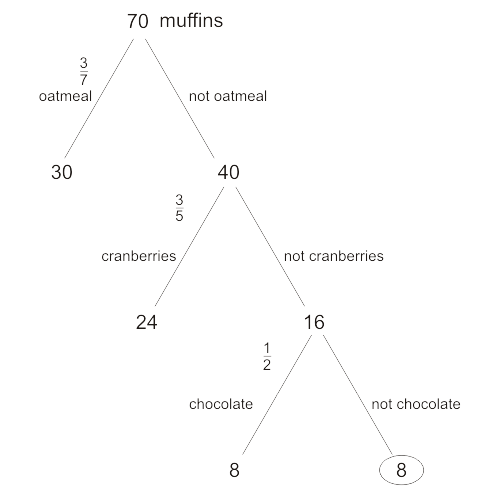We cover every section of the GMAT with in-depth lessons, 5000+ practice questions and realistic practice tests.

## Up to 100+ points GMAT score improvement guarantee

### The best guarantee you’ll find

Our Premium and Ultimate plans guarantee up to 100+ points score increase or your money back.

## Master each section of the test

### Comprehensive GMAT prep

We cover every section of the GMAT with in-depth lessons, 5000+ practice questions and realistic practice tests.

## Schedule-free studying

### Learn on the go

Study whenever and wherever you want with our iOS and Android mobile apps.

# Plugging In: Invisible Plugging In - Fractions

In a bakery shop $$\frac{3}{7}$$ of the muffins are oatmeal, and $$\frac{3}{5}$$ of the remaining muffins are cranberry muffins. Of the muffins that are neither oatmeal nor cranberries, $$\frac{1}{2}$$ are plain chocolate muffins. What fraction of all the muffins are neither oatmeal, cranberries, nor plain chocolate?
Correct. [[snippet]] Assume that there are 70 muffins in the bakery shop. Model the problem using a tree:Of the total muffins, $${3}{7}$$ of them are oatmeal, so there are >$$\frac{3}{7}\times 70 = 3 \times 10 = 30$$ oatmeal muffins. Of the remaining 40 muffins, $$\frac{3}{5}$$ are cranberries, so there are >$$\frac{3}{5}\times 40 = 3\times 8 = 24$$ cranberry muffins. Of the remaining $$40-24=16$$ muffins, half are plain chocolate. So there are 8 plain chocolate muffins with 8 more remaining muffins that are not members of either of the categories we've dealt with so far. That means that the fraction of muffins that are not oatmeal, cranberry, or plain chocolate is $$\frac{8}{70}$$. Reduce that fraction by 2 to get $$\frac{4}{35}$$.
Incorrect. [[snippet]]
Incorrect. [[snippet]]
Incorrect. [[snippet]] This is the fraction of muffins that *are* oatmeal, cranberries, or plain chocolate. But what did the question ask?
Incorrect. [[snippet]]
$$\frac{1}{70}$$
$$\frac{4}{35}$$
$$\frac{18}{70}$$
$$\frac{52}{70}$$
$$\frac{31}{35}$$